World-class research. Ultimate impact.
More on impact ›

# Frontiers in Astronomyand Space Sciences## Original Research ARTICLE

Front. Astron. Space Sci., 12 April 2019 | https://doi.org/10.3389/fspas.2019.00022

# Coronal Loop Seismology Using Standing Kink Oscillations With a Lookup Table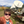David J. Pascoe1*,Alan W. Hood2 and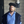Tom Van Doorsselaere1
• 1Department of Mathematics, Centre for Mathematical Plasma Astrophysics, KU Leuven, Leuven, Belgium
• 2School of Mathematics and Statistics, University of St Andrews, St Andrews, United Kingdom

The transverse structure of coronal loops plays a key role in the physics but the small transverse scales can be difficult to observe directly. For wider loops the density profile may be estimated by forward modeling of the transverse intensity profile. The transverse density profile may also be estimated seismologically using kink oscillations in coronal loops. The strong damping of kink oscillations is attributed to resonant absorption and the damping profile contains information about the transverse structure of the loop. However, the analytical descriptions for damping by resonant absorption presently only describe the behavior for thin inhomogeneous layers. Previous numerical studies have demonstrated that this thin boundary approximation produces poor estimates of the damping behavior in loops with wider inhomogeneous layers. Both the seismological and forward modeling approaches suggest loops have a range of layer widths and so there is a need for a description of the damping behavior that accurately describes such loops. We perform a parametric study of the damping of standing kink oscillations by resonant absorption for a wide range of inhomogeneous layer widths and density contrast ratios, with a focus on the values most relevant to observational cases. We describe the damping profile produced by our numerical simulations without prior assumption of its shape and compile our results into a lookup table which may be used to produce accurate seismological estimates for kink oscillation observations.

## 1. Introduction

Coronal loops are modeled as density structures in the solar atmosphere which act as waveguides for several types of magnetohydrodynamic (MHD) modes. One of the most readily detectable are standing kink oscillations, which causes periodic transverse perturbations of the loop axis. These oscillations were first detected using the Transition Region And Coronal Explorer (TRACE; Aschwanden et al., 1999, 2002; Nakariakov et al., 1999). The number of observations has greatly increased since the launch of the Atmospheric Imaging Assembly (AIA; Lemen et al., 2012) onboard the Solar Dynamics Observatory (SDO). Catalogs of observations have been produced by Zimovets and Nakariakov (2015) and Goddard et al. (2016). Kink oscillations attract a lot of attention due to their potential for seismological studies of the coronal plasma (e.g., reviews by De Moortel and Nakariakov, 2012; Stepanov et al., 2012; Pascoe, 2014; De Moortel et al., 2016), in particular to estimate the strength of the coronal magnetic field (e.g., Nakariakov et al., 1999; Nakariakov and Ofman, 2001; Van Doorsselaere et al., 2007; White and Verwichte, 2012; Pascoe et al., 2016; Sarkar et al., 2016).

Kink oscillations are observed to be strongly damped, only having a detectable amplitude for a few cycles, which is attributed to resonant absorption (e.g., Chen and Hasegawa, 1974). Coronal loops are modeled as having a higher density than the surrounding plasma, and for resonant absorption to take place only requires that the transition between the higher and lower density plasma is smooth. Inside this inhomogeneous layer, wave energy is transferred from kink to Alfvén modes where the local Alfvén speed matches the kink speed Ck. The timescale of this process is comparable to the period of oscillation (e.g., Hollweg and Yang, 1988; Goossens et al., 1992, 2002; Ruderman and Roberts, 2002).

Resonant absorption is a robust mechanism which occurs even in loops which are not cylindrically symmetric (Terradas et al., 2008b; Pascoe et al., 2011). Furthermore, numerical studies demonstrate that the Kelvin-Helmholtz instability (e.g., Terradas et al., 2008a; Antolin et al., 2015; Okamoto et al., 2015; Magyar and Van Doorsselaere, 2016; Hillier et al., 2019) is most efficient in loops with thin inhomogeneous layers. This instability leads to a mixing of plasma and effective widening of the inhomogeneous layer (e.g., Goddard et al., 2018; Karampelas and Van Doorsselaere, 2018) in addition to increased heating due to phase mixing (e.g., Heyvaerts and Priest, 1983; Karampelas et al., 2017; Pagano et al., 2018; Guo et al., 2019) which can account for observations of broad differential emission measures in coronal loops (Van Doorsselaere et al., 2018).

The transverse density profile can be described by two dimensionless parameters; the density contrast ratio being the ratio of the density at the center of the loop to the density far from it ζ = ρ0e, and the width of the inhomogeneous layer normalized to the minor loop radius ϵ = l/R. The damping rate due to resonant absorption depends on both of these parameters. For this reason the problem is underdetermined when trying to infer ζ and ϵ from the (exponential) damping time alone and some additional information is required (e.g., Goossens et al., 2008; Arregui and Asensio Ramos, 2014; Arregui and Goossens, 2019). Pascoe et al. (2013) produced a more accurate description of the damping profile due to resonant absorption, which includes the initial Gaussian damping regime of kink oscillations in addition to the later exponential damping regime. This damping profile is characterized by two damping times and so allows both ζ and ϵ to be estimated. This method was first applied by Pascoe et al. (2016) and later extended by Pascoe et al. (2017a,c) to include additional effects such as a time-dependent period of oscillation, the presence of additional parallel harmonics, and the use of Bayesian analysis (e.g., Arregui et al., 2013; Arregui, 2018) to improve the estimation of uncertainties. This seismological method requires both the Gaussian and exponential damping regimes to be accurately detected in the data and so depends on the oscillation data having a sufficiently high quality.

Another method for estimating ϵ is by forward modeling the appearance of the density profile for direct comparison with the transverse EUV intensity profile of the loop (Goddard et al., 2017; Pascoe et al., 2017b). It is possible to apply both of these methods simultaneously to observational data. This was recently demonstrated by Pascoe et al. (2018) for a loop for which the oscillation data alone was too noisy to allow strong constraint of ζ and ϵ. However, the spatial information from the intensity profile produced a strong constraint on ϵ, such that the oscillation data was only required to infer ζ when both methods were applied simultaneously. On the other hand, the value of ϵ ≈ 0.9 for this observation is significantly outside the validity of the thin boundary (TB) approximation. To correct for this effect, Pascoe et al. (2018) performed a narrow parametric study using the TB estimate as a starting point. The result of this study was a change in the estimated value of ζ from the TB value of 2.3 to a value of 2.8 based on numerical simulations for ϵ = 0.9. This case demonstrates the need for a seismological method that can account for the behavior of kink oscillations in loops with wide inhomogeneous layers without the need for separate studies and corrections applied afterwards. The use of a self-consistent seismological method is particularly important for future development of techniques for data analysis where multiple observational signatures are forward modeled and a systematic error arising from the TB approximation would have a deleterious influence on other observables. For example, the EUV intensity is I∝ρ2 and so a change in inferred density contrast from 2.3 to 2.8 in the example above corresponds to an intensity change by a factor of approximately 1.5.

In this paper we study the behavior of kink oscillations of coronal loops for various transverse density profiles. Our aim is to provide a simple method of estimating the damping profile for a chosen profile which may be used for seismological investigations. The damping profiles for resonant absorption used in previous studies and this one are described in section 2. The results of our parametric study and the generation of a lookup table (LUT) are presented in section 3. In section 4 we present examples of the application of our method to synthetic test cases and observational data. Conclusions are presented in section 5.

## 2. Damping Profile for Kink Oscillations

Initial applications of resonant absorption to explain the strong damping of kink oscillations (Goossens et al., 1992, 2002; Ruderman and Roberts, 2002) considered only the asymptotic state of the system. The damping profile was an exponential of the form

where τd is the exponential damping time, P is the period of oscillation, κ = (ρ0−ρe)/(ρ0e) and the factor 4/π2 corresponds to a linear transition between ρ0 and ρe. In this paper we consider a linear profile for the density in the inhomogeneous layer since it is the simplest smooth profile, can describe the widest range of possible structures, and is the only profile for which the analytical solution for all times is presently known (see discussion section 6.2 of Pascoe et al., 2018).

Numerical simulations by Pascoe et al. (2012) demonstrated that the damping profile of strongly damped propagating kink oscillations is more accurately described by a Gaussian damping profile rather than an exponential one. The existence of these two regimes was reconciled by the analytical description derived by Hood et al. (2013) which described the damping profile for all times and demonstrates that Gaussian and exponential profile can be obtained in the limits of small and large time, respectively. The derivation by Hood et al. (2013) was performed for propagating kink waves with the damping rate expressed in terms of damping length scales but we may consider the case of standing kink waves with a damping time using the long wavelength limit λ = CkP, giving

We note that the relationship between damping length scales (propagating waves) and timescales (standing waves) has been demonstrated explicitly for the exponential regime (e.g., comparing the derivations of Goossens et al., 2002; Terradas et al., 2010) but presently not the Gaussian regime. Nonetheless we demonstrate the applicability of this relationship (proposed by Pascoe et al., 2010) by comparison with our numerical simulations. The applicability of this relationship has also previously been demonstrated in numerical simulations by Ruderman and Terradas (2013) and Magyar and Van Doorsselaere (2016).

Pascoe et al. (2013) proposed a general damping profile (GDP) that combined both of these damping regimes into a single approximation. This is described in terms of a switch from the Gaussian damping profile that applies at the start of the oscillation to the exponential profile which applies later.

where the switch from the Gaussian to exponential damping regime occurs at ts and ${A}_{\text{s}}={D}\left(t={t}_{\text{s}}\right)$.

The above damping profiles are also based on the thin tube approximation. In this limit the period of the fundamental standing kink mode is

where L is the loop length, and the kink speed for a low-β plasma (uniform magnetic field) is

where CA0 is the internal Alfvén speed. The thin tube thin boundary (TTTB) approximation for the period of oscillation of kink modes therefore depends on ζ but not ϵ (Goossens et al., 2008). However, the parametric studies by Van Doorsselaere et al. (2004) and Soler et al. (2014) find that Pk does depend on ϵ outside of the applicability of the TB approximation.

To illustrate the different damping profiles, Figure 1 shows the results of our numerical simulations (described in section 3) for three values of ϵ with ζ = 2. For this value of density contrast the GDP suggests a switch from the Gaussian to exponential damping regime at t = 3P. For kink oscillations in low density contrast loops such as these, the Gaussian damping profile (blue curves) provides a much better description than the exponential damping profile (red curves), and the general damping profile (green curves) further improves the description for later times. As expected, all three analytical profiles become poorer as ϵ increases and the TB approximation becomes less appropriate.

FIGURE 1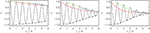Figure 1. Comparison of kink oscillations calculated by numerical simulations (solid lines) with the analytical damping profiles (colored lines). The red, blue, and green lines correspond to the exponential, Gaussian, and general damping profiles, respectively. The plus symbols represent the amplitudes used to characterize the oscillation in our lookup table (LUT). The LUT damping profiles (dashed lines) are constructed from these amplitudes by spline interpolation. The left, middle, and right panels show the results for ϵ = 0.1, 0.5, and 1.0, with ζ = 2 for all cases.

In this paper we wish to characterize the damping behavior of kink oscillations as accurately as possible, and without prior assumption of the form of the damping profile. The plus symbols in Figure 1 represent the amplitudes Ai which we use to characterize the oscillation. These amplitudes are defined at every half cycle of the oscillation. The LUT damping profile (dashed lines) is constructed from these amplitudes by spline interpolation. The dashed lines indicate that this method allows us to accurately describe the damping of the kink oscillation, albeit at a cost of requiring more information. The exponential and Gaussian damping profiles can each be characterized by a single parameter, i.e., the damping time τd or τg. The GDP combines both the Gaussian and exponential damping regimes and so is characterized by both these damping times (with the switch time given in terms of these two parameters in Equation 6). For the six cycles indicated in Figure 1 our interpolation method uses 13 parameters, or more generally 2n+1 parameters for n cycles. On the other hand, this number is still sufficiently small that the results of hundreds of numerical simulations can be compiled into a lookup table of minimal size.

For each of the three simulations in Figure 1, and the additional simulations presented in section 3, the amplitudes Ai used to describe the LUT damping profile were found by a least-squares fit to the data using MPFIT (Markwardt, 2009). Simulation data covered at least six cycles of the oscillation, with a greater number for simulations with smaller values of ζ or ϵ for which the damping is weaker and so there are a greater number of cycles at an observable amplitude. This is also intended to ensure that the exponential damping regime is present in the data, which occurs later for smaller ζ. This allows the damping profile to be extrapolated beyond the number of simulated cycles using an exponential profile with the last measured damping time. We require that the LUT damping profile function is able to return results for arbitrary values of time in order for it to appropriately handle requests from the user or from a fitting routine transparently i.e., without needing to take the details of individual simulations into account.

## 3. Parametric Study

In this section we study the behavior of kink oscillations in coronal loops for various combinations of ζ and ϵ. Soler et al. (2014) and Van Doorsselaere et al. (2004) performed similar numerical studies investigating the damping time for the exponential regime. These studies demonstrated that the thin boundary approximation produces poor estimates of the damping behavior in loops with wider inhomogeneous layers.

As in Soler et al. (2014), we consider L/R = 100 which is typical for observations of standing kink oscillations. Weak dependence of the damping on the longitudinal wavenumber kz has been demonstrated by Van Doorsselaere et al. (2004). Numerical simulations were performed using a Lax-Wendroff code to solve the linear MHD equations in cylindrical coordinates (r, θ, z) for m = 1 symmetry corresponding to kink oscillations (and the Alfvén waves generated by resonant absorption). The magnetic field is constant and aligned with the z-direction. This code was previously used in Pascoe et al. (2012, 2013, 2015) and Hood et al. (2013) to study the spatial damping of propagating kink waves and here is applied to the case of the temporal damping of standing kink waves by appropriate choice of boundary and initial conditions. The boundary conditions are line-tied to simulate the loop footpoints being fixed in the photosphere, while the boundary in the r-direction is placed sufficiently far away to not affect the results. The fundamental longitudinal harmonic of the standing kink mode is excited by a perturbation to the radial and azimuthal velocities of the form

In the following simulations, the numerical domain covers r = [0, 10R] and z = [0, 100R], with a resolution of 1, 000 × 1, 000 grid points. Each of our 300+ simulations took approximately 1 h to run using 80 × 2.8 GHz processor cores. We study the standing mode by considering the variation in the amplitude of the transverse velocity measured at the loop apex z = L/2.

Figure 2 shows the simulations performed in our parametric study which were used to generate the first version of our lookup table. The solid, dashed, and dotted lines correspond to the damping rates shown in the right panel. These damping profiles are based on the TB approximation for the Gaussian damping profile and so will not accurately describe the behavior for large ϵ but serve as an indication of the range of parameters we are interested in considering with respect to observational studies. The curves demonstrate that we are not equally interested in all regions of the ζ-ϵ parameter space. Large amplitude standing kink oscillations are typically observed for fewer than 6 cycles (e.g., Goddard and Nakariakov, 2016) and so we are mainly interested in parameters which produce this level of damping. The dotted, solid, and dashed lines correspond to τg/P = 1, 2, and 5, respectively, and indicate the parameters we expect to be relevant to observations. For large values of both ζ and ϵ, kink oscillations would be very strongly damped and hence unlikely to be reliably detected. The shorter time series available would also generally limit the seismological information that could be obtained. Unlike ϵ which has the defined upper limit of 2, there is no upper limit for ζ. However, the damping rate is asymptotic in this limit and so we can consider a reasonable upper limit, which is taken to be 7 in this study but may be extended in the future (e.g., high contrast filament threads considered by Arregui et al., 2008). For small values of both ζ and ϵ the oscillations would be weakly damped. Such oscillations are not typically observed, although we are still interested in the behavior for small values of ϵ with regard to checking convergence of our results to the analytical profiles based on the TB approximation.

FIGURE 2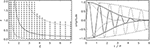Figure 2. The black circles denote the 300 numerical simulations that comprise version 1.0 of our LUT. The solid, dashed, and dotted lines correspond to the damping rates shown in the right panel. These damping profiles are based on the TB approximation for the Gaussian damping profile but serve as an indication of the range of parameters we are interested in considering with respect to observational studies.

The distribution of the simulations we have performed reflects these areas of interest. When compiling the results of these simulations into a lookup table, it is therefore not convenient to use a 2D array to describe the ζ-ϵ plane. Instead, the results are considered as a list of scattered positions in the parameter space using the IDL routine GRIDDATA which can be used to interpolate our simulation results to return the damping profile for an arbitrary value of ζ and ϵ. Additional simulations were performed for testing purposes, including generating synthetic observational data used in section 4. These additional simulations are not included version 1.0 LUT but may be in future applications of the LUT to actual observational data. The use of a LUT and interpolation methods for scattered data allows the method to be improved over time by incorporating additional results as they are obtained. Other examples of a LUT strategy for solar applications include the CHIANTI emission database (Del Zanna et al., 2015) used as part of EUV forward modeling codes such as FoMo (Van Doorsselaere et al., 2016), and the CAISAR code for inversions of solar Ca II spectra (Beck et al., 2015).

The LUT and the corresponding IDL code are available at https://github.com/djpascoe/kinkLUT. The routine requires as input the values of ϵ, ζ, and the normalized times tn = t/P at which the damping profile is desired. The value of each amplitude Ai is determined by 2D interpolation of the simulation results using the IDL routine GRIDDATA. (In this paper we use the linear method, chosen as the simplest method with fewest assumptions, for which requested grid points are linearly interpolated from triangles formed by Delaunay triangulation. These triangles were constructed with the TRIANGULATE routine and are included in the LUT save file). The damping profile is then returned by spline interpolation of these amplitudes Ai for the user-requested times tn. This procedure can be used within a forward modeling function used for comparing a model with data. For example, a simple model for an oscillation with a single harmonic and no background trend, as considered in this paper, is

where A0 is the initial amplitude of a sinusoidal oscillation with period P and phase shift ϕ, and the damping profile ${{D}}_{LUT}$ is based on our lookup table. We demonstrate the results of such a method in section 4.

### 3.1. Dependence of Period of Oscillation and Damping Rate on Transverse Density Profile

Here we compare the results of our parametric study with the analytical profiles discussed in section 2 and previous numerical studies.

Figure 3 shows the dependence of the kink mode behavior on ζ. For an exponential or Gaussian damping profile it is convenient to characterize the damping with the damping time (or length scale for propagating waves). However, in this study we make no assumption about the shape of the damping profile and so we consider the damping which has occurred after a certain time, or a certain number of oscillation cycles since the period of oscillation also depends on ζ. The colored lines correspond to the theoretical damping rates based on the TB approximation and an exponential (red), Gaussian (blue), or general (green) damping profile.

FIGURE 3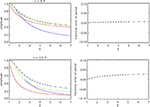Figure 3. Dependence of the damping of the kink oscillation on the density contrast ratio ζ. The top panels show results for ϵ = 0.1 and the bottom panels for ϵ = 0.5. The left panels show the kink oscillation amplitude (plus symbols) taken at a fixed time (t = 5P or 2.5P) The colored lines show the estimates based on the general damping profile (green), Gaussian damping profile (blue), and exponential damping profile (red). The right panels show the variation of the period of oscillation compared with the theoretical value Pk.

The top panels of Figure 3 demonstrate the case of a thin inhomogeneous layer (ϵ = 0.1) where the TB approximation is appropriate. The top left panel reproduces the known dependence of the shape of the damping profile on ζ. For lower density contrasts the Gaussian profile better describes the damping. The GDP which combines both profiles, with a switch from Gaussian to exponential that depends on ζ, provides a significantly better approximation for all values of ζ. The switch time occurs at 5P for ζ = 1.5 and so the general and Gaussian damping profiles are identical for ζ ≤ 1.5.

The bottom panels of Figure 3 demonstrate the case of a finite inhomogeneous layer (ϵ = 0.5) where the TB approximation is less appropriate. The estimated period of oscillation is still reasonable but the damping is being significantly overestimated. The amplitude is taken at the earlier time of 2.5P since the damping is stronger for the larger value of ϵ. At this earlier time, the Gaussian damping profile is always a better approximation than the exponential profile for the range of ζ ≤ 7 considered. The switch time occurs at 2.5P for ζ ≈ 2.3, and so it is expected that the Gaussian estimate is better than the exponential estimate for density contrasts lower than this value. However, it also remains a better overall estimate for contrasts not too much above this value due to the exponential estimate being so poor during the first cycle or so. The Gaussian estimate therefore does not become poorer than the exponential estimate by the time of 2.5P considered in the bottom panel of Figure 3, whereas it does in the top panel. Whether the Gaussian or exponential estimate is better therefore depends on not only when the switch occurs but also how much data is considered after that switch. The general damping profile provides the best approximation for all parameters and times, but is also inaccurate when there is significant damping due to the limitations of the TB approximation it is based upon.

The right panels of Figure 3 show the fractional error in the period of oscillation estimated as Pk by Equation (7). The errors are typically very small since the thin tube approximation (ω/kz = Ck) is appropriate for our simulations with L/R = 100. The error increases with ζ due to the stronger dispersion present in higher contrast loops, and is also found to increase with ϵ (see also Figures 4, 6).

FIGURE 4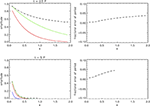Figure 4. Dependence of the damping of the kink oscillation on the inhomogeneous layer width ϵ. The top panels show results for ζ = 2 and the bottom panels for ζ = 7. The left panels show the kink oscillation amplitude (plus symbols) taken at a fixed time (t = 5P or 2.5P) The colored lines show the estimates based on the general damping profile (green), Gaussian damping profile (blue), and exponential damping profile (red). The right panels show the variation of the period of oscillation compared with the theoretical value Pk.

Figure 4 shows the dependence of the kink mode behavior on ϵ. The top panels show results for ζ = 2 at t = 2.5P, i.e., the behavior at an early time for a low density contrast ratio. For ζ = 2 the switch for Gaussian to exponential occurs at t = 3P and so the Gaussian and general damping profiles are identical before this time, and are a significantly better approximation than the exponential profile. The bottom panels show results for ζ = 7 at t = 5P, i.e., having both a sufficiently high density contrast and a sufficiently large number of cycles for the exponential damping profile to always be a better approximation than the Gaussian profile, though the GDP remains an improvement over both.

Figure 5 shows 2D contours for the amplitude of the kink oscillation at t = 3P (top left panel) and the fractional errors of the corresponding estimates based on the TB approximation. The errors tend to zero in the appropriate limit ϵ → 0, otherwise each approximation underestimates the amplitude. The Gaussian and GDP estimates are also accurate in the limit ζ → 1 since they describe the initial stage of resonant absorption, whereas the exponential estimates remain poor in this limit when ϵ > 0.

FIGURE 5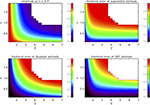Figure 5. Amplitude of the kink oscillation at t = 3P (top left) and the fractional errors of the corresponding estimates based on the TB approximation and an exponential (top right), Gaussian (bottom left), or the general (bottom right) damping profiles.

Figure 6 shows the fractional error in the period of oscillation estimated using the TB approximation (Equation 7). The TB approximation underestimates the period of oscillation. The dependence of the error on ζ and ϵ is similar to that found by Soler et al. (2014) (i.e., being proportional to the strength of the damping due to resonant absorption) but the magnitude is smaller, remaining less than 6%. Soler et al. (2014) report an error of up to 45% in their study which considers ζ up to 20, whereas we restrict our attention to the parameter range most relevant to observations (e.g., ζ ≤ 3.5 for the largest values of ϵ in Figure 2). However, even accounting for this there remains a discrepancy and Soler et al. (2014) find errors greater than 30% for a comparable parameter range.

FIGURE 6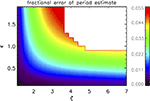Figure 6. Error in the period of oscillation estimate based on the TB approximation as given by Equation (7).

Figure 7 shows the difference in fractional error using the exponential damping profile alone compared with the GDP based on Figure 5. It indicates the GDP analysis is always an improvement over the exponential profile, and the difference is largest for lower ζ, typically ~60% for ζ < 2 and ~30% for ζ>2. The right panel of Figure 7 shows how the fractional error (averaged over 300 numerical simulations) varies as a function of time for each of the profiles based on the TB approximation. Each of the errors increase in time due to the cumulative effect of overestimating the damping rate, however the GDP remains at all times a significantly better estimate of the kink oscillation amplitude. The GDP (Equation 6) is a simple modification of the exponential damping profile with no additional parameters and so this improved estimate comes at effectively no computational cost. Our LUT method is based on several interpolation routines and so is slower to calculate than the GDP but remains practical.

FIGURE 7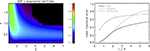Figure 7. Difference between the fractional error using the exponential damping profile alone compared with the GDP (left), and the mean fractional error as a function of time for each of the three TB profiles (right).

The larger errors for analysis based on the exponential profile arise because it provides a very poor description for the initial behavior of kink oscillations. Pascoe et al. (2013) demonstrate that the seismological estimate based on the exponential profile is significantly improved by ignoring the first two cycles of the oscillation and only analysing the remaining data. However, this is not a practical solution for detailed analysis of oscillations since it means the initial amplitude cannot be estimated, which is important for nonlinear effects. It would also hinder the potential to detect higher harmonic oscillations which have a shorter period and so typically only exist for the first few cycles of the fundamental mode (e.g., Pascoe et al., 2017a). For example, if the fundamental mode is observable for six cycles then the third harmonic with P3P1/3 but the same damping per period would only be detectable during the first two cycles of the fundamental mode.

## 4. Seismological Application

In this section we demonstrate the application of our LUT as a seismological tool to use the observed damping of a kink oscillation to infer information about the transverse density profile of the oscillating loop.

Figure 8 shows the results of a test of our method for a kink oscillation simulated in a loop with ζ = 2.15 and ϵ = 0.75. This data point is not included in version 1.0 of our LUT used in the following analysis. The top panel shows the analyzed oscillation which includes uniformly distributed random noise with a maximum amplitude of 5% of the initial kink oscillation amplitude. The middle and bottom panels show 2D histograms approximating the marginalized posterior probability density function for ζ and ϵ based on 105 Markov chain Monte Carlo (MCMC) samples of the GDP and LUT models, respectively (see also Pascoe et al., 2017a, 2018). This data comes from a simulation with an inhomogeneous layer width that is sufficiently large for the TB approximation to produce inaccurate results. The GDP approach overestimates the value of ϵ, and correspondingly underestimates the value of ζ.

FIGURE 8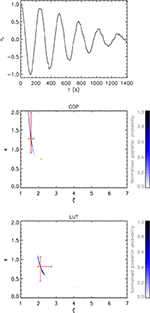Figure 8. The top panel shows a test kink oscillation simulated for ζ = 2.15 and ϵ = 0.75 with noise added. The middle and bottom panels show the seismologically estimated transverse density structure based on the GDP and LUT methods, respectively. The color contours represent the normalized 2D histograms approximating the marginalized posterior probability density function. The red error bars correspond to the MAP value and 95% credible intervals, and the green circle indicates the actual density profile parameters.

The seismological estimates in Figure 8 demonstrate that it is important to consider the dependence of the damping on ζ and ϵ, aside from the quality of the observational data. Due to the asymptotic nature of the inversion curve, the extent to which ζ and ϵ are constrained depends on whether the density contrast is high or low; near the low-ζ asymptote ζ is well-constrained but ϵ is poorly constrained, and vice versa for the high-ζ asymptote. This is demonstrated in Figure 8 where the GDP inversion underestimates the actual value of ζ = 2.15 and produces very low estimates of ζ. The red bars indicate the 95% credible intervals and show the value of ζ is well-constrained (but excludes the actual value of ζ due to the systematic error from the TB approximation). In comparison, the inversion results using the LUT are less constrained for ζ but the maximum a posteriori probability (MAP) estimate of ζ ≈ 2.11 is consistent with the actual value for the synthetic data. The nature of these constraints makes 2D histograms such as those in Figure 9 the simplest way of representing the available information for the transverse density profile. Quoting confidence intervals alone can be misleading if the asymptotic behavior is not kept in mind. Furthermore, 1D histograms for ζ and ϵ would generally not be well described using a normal distribution (they have been approximated by the exponentially modified Gaussian function by Pascoe et al., 2017a) and so estimates of uncertainties based on assuming this distribution would not be accurate.

FIGURE 9

Figure 9 shows the results of a test of our method for a loop with a thin boundary layer ζ = 3.75 and ϵ = 0.15 (this data point is again not included in version 1.0 of our LUT). In this limit we see both the GDP and LUT inversions producing good estimates of the actual value of ϵ. The asymptotic behavior means ζ is far less constrained than ϵ, and less constrained for the LUT results since the inferred value of ζ is larger (it is underestimated using the GDP).

### 4.1. Comparison With Previous Spatiotemporal Analysis

Pascoe et al. (2018) presented a method for spatiotemporal analysis of coronal loops which used both the transverse EUV intensity profile and the standing kink oscillation to constrain the properties of the coronal loop. In that work the spatial analysis (forward modeling of the transverse EUV profile) provided a value of ϵ ≈ 0.9 and so a narrow parametric study for that particular observation was performed to estimate the effect of a thick inhomogeneous layer on the seismological component of their analysis. Here we demonstrate how the results may be reproduced from the much wider parametric study in this paper.

Figure 10 shows the results of applying our method to the oscillation of Leg 1 as previously analyzed. We consider the prior for ϵ with the form of a normal distribution with a mean of 0.9 and standard deviation of 0.1 (e.g., Figure 4 of Pascoe et al., 2018). The seismological analysis of the oscillation then proceeds as in section 4.1 of Pascoe et al. (2018), with results based on 106 MCMC samples of a model consisting of a damped sinusoidal oscillation which begins at a start time t0 ≈ 4 minutes and having a background trend described by spline interpolation using six reference points across the time series. We consider both the GDP and LUT damping profiles. The top panels in Figure 10 show the loop position (plus symbols) with the color contour representing the normalized posterior predictive probability density for each damping profile model. The GDP (left panels) results infer a value of $\zeta =2.{1}_{-0.2}^{+0.4}$ (based on the MAP value and 95% credible interval) consistent with the previous analysis. The narrow parametric study in section 6.4 of Pascoe et al. (2018) suggested ζ ≈ 2.8 as an improved estimate, correcting for the TB approximation used in the GDP. The right panels of Figure 10 show our analysis using the LUT damping profile, which infers $\zeta =2.{9}_{-0.5}^{+0.9}$, consistent with that estimate. The Bayes factor (e.g., Jeffreys, 1961; Kass and Raftery, 1995) for the LUT model compared with the GDP is K = 1.5, i.e., too small to distinguish which model is better based on their reproduction of the data alone. This is in contrast to the very strong Bayesian evidence for the GDP compared with an exponential damping profile found in Pascoe et al. (2018). For this data, the shape of the damping profile produced by the LUT is very similar to the shape produced by the GDP (but significantly different to an exponential damping profile), though the inferred values of ζ and ϵ that correspond to that shape differ. Considering that ϵ ≈ 0.9 whereas the GDP is based on the TB approximation, the LUT method is more appropriate on the basis of self-consistency. The GDP underestimates the LUT density contrast ratio by approximately 25%, and analysis using an exponential damping profile underestimates ζ by approximately 50%.

FIGURE 10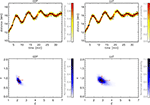Figure 10. Analysis of the oscillation reported in Pascoe et al. (2018) using the GDP and LUT damping profile. The top panels show the position of Leg 1 (plus symbols) with the color contour representing the normalized posterior predictive probability density for each damping model. Bottom panels as in Figure 8.

The observation considered by Pascoe et al. (2018) was one for which the spatial analysis provided a well-constrained value of ϵ, independent of the seismological analysis. It was therefore possible in that study to consider the correction to the TB approximation in terms of the dependence on ζ alone, requiring only a narrow 1D parametric study for the estimate. The LUT for the 2D parametric study in this paper allows the method in Pascoe et al. (2018) to be extended to a greater number of loops where the spatial information might not be as conclusive on its own but can assist in further constraining the seismologically-inferred parameters.

## 5. Conclusions

In this paper we have performed a parametric study for the dependence of the damping of standing kink oscillations by resonant absorption on the density contrast ratio ζ and the width of the inhomogeneous layer ϵ. Previous studies (Van Doorsselaere et al., 2004; Soler et al., 2014) have demonstrated the inaccuracy of the classical analytical description for the damping rate τd, which is based on the TB approximation, when applied to loops with large values of ϵ. We have expanded on these studies by developing a description of the damping profile which includes the initial non-exponential regime, and summarized our results into a lookup table which may be used to produce improved estimates of loop parameters.

The method presented in this paper makes analysis simpler in terms of not requiring an additional step to correct for the effect of a wide inhomogeneous layer, as in Pascoe et al. (2018), and automatically propagates the corresponding uncertainties. Being self-consistent also makes the method more suitable than the TB approximation as a starting point to include other effects which depend on the density profile parameters. On the other hand, this work only accounts for the influence of wide inhomogeneous layers on the kink oscillation damping profile, with other assumptions and approximations needing to be considered.

The simulations performed in this paper use a linear profile for the density in the inhomogeneous layer. In terms of the damping rate due to resonant absorption, the key physical parameter is the gradient of the Alfvén speed at the resonance location. Arregui and Goossens (2019) discuss how the choice of density profile (e.g., linear or sinusoidal in the inhomogeneous layer) affects the seismologically-inferred loop parameters, since they have different constants of proportionality (e.g., Goossens et al., 2002; Roberts, 2008; Soler et al., 2014) between the damping time and the loop parameters (ζ, ϵ). Arregui and Goossens (2019) note that seismological applications have so far used the linear profile and that choosing a different profile would return a different answer. However, presently no such choice exists since the linear profile is the only one for which a full analytical solution is known (Hood et al., 2013; Ruderman and Terradas, 2013). The linear profile is therefore the natural choice of profile for use in this study, to allow a comparison of numerical results with the TB approximations in the limit ϵ → 0. Pascoe et al. (2018) estimate that the difference in the seismological results using the linear and sinusoidal density profiles is ≲10%. However, these two profiles are not the only possible choices. If some profile (other than the linear one used) should be shown to be more appropriate for coronal loops then it would be simple (though computationally expensive) to reproduce our parametric study for that profile. If the spatial resolution of loops is sufficiently high then it is possible to test different profiles by forward modeling the EUV profile (e.g., Aschwanden et al., 2007; Goddard et al., 2017; Pascoe et al., 2017b, 2018). Such methods still typically involve further assumptions or approximations (e.g., loops having a cylindrical cross-section and azimuthal symmetry) however the aim of these investigations should not be considered the impossible task of inferring the precise density profile of coronal loops but rather estimating the most appropriate characteristic scales that influence the relevant physical processes. Resonant absorption, phase mixing, and the Kelvin-Helmholtz instability all depend on the transverse loop structure. In this context it is not required that the coronal loop density profile is exactly linear for the analysis based on this profile (or any other simplified model) to provide accurate and useful estimates of parameters such as the size of the transverse inhomogeneity l = ϵR.

Our LUT is also based on results of numerical simulations for the linear regime and so excludes the effect of the Kelvin-Helmholtz instability (KHI). The current technique is therefore more suitable for loops with wider inhomogeneous layers in which KHI develops at a slower rate. Simulations by Magyar and Van Doorsselaere (2016) suggest KHI is weak for ϵ≳0.5 and the weaker development for larger values of ϵ is also evident in simulations by Goddard et al. (2018) and Pagano et al. (2018). In principle the same LUT strategy could be extended to include effects such as KHI by performing a similar parametric study with a third parameter being the amplitude of the oscillation, A0, with the study in this paper representing the ζ-ϵ plane in the limit A0 → 0. However this wider parametric study (ζ, ϵ, A0) where each data point is based a 3D nonlinear simulation would be orders of magnitude more computationally expensive than our current one. A wider parametric study which describes the evolution of the loop, e.g., the development of fine structure by KHI, would also require additional observable signatures to be modeled and compared with data. In the current study we focus on the shape of the damping profile due to resonant absorption. Additional observational signatures have recently been considered by Antolin et al. (2017) based on forward modeling techniques (Van Doorsselaere et al., 2016) to describe the appearance of loops observed simultaneously in multiple EUV channels.

Our parametric study was also performed for thin loops which satisfy the long wavelength approximation kzR≪1. As a first approximation the effect of a large kz may be considered in terms of reducing the kink mode phase speed from Ck, but otherwise not influencing the damping per cycle (Van Doorsselaere et al., 2004). Variations in the loop length in time can account for a time-dependent period of oscillation (e.g., Nisticò et al., 2013; Russell et al., 2015; Pascoe et al., 2017c) but should also remain well-approximated by considering the damping per cycle provided by our LUT as unaffected. The period of oscillation (and ratios of periods for different longitudinal harmonics) can also be affected by longitudinal structuring due to gravity (e.g., Andries et al., 2005). Cooling of coronal loops causes the scale height to vary in time, accompanied by decreases in the period and period ratio (Morton and Erdélyi, 2009) and an amplification of kink oscillation which acts against the damping due to resonant absorption (Ruderman, 2011). Numerical simulations suggest this effect is typically small but could be approximately 10% for larger amplitude oscillations (Magyar et al., 2015). Similarly, the period of oscillation and period ratio can be affected by expansion of the loop at the apex (Verth and Erdélyi, 2008) and a time-dependent expansion can also reduce the damping of kink oscillations (Shukhobodskiy et al., 2018). It may be possible to include effects such as these which modify the period of oscillation relative to Pk and introduce additional damping/amplification terms into a more general oscillation model alongside our LUT damping profile for resonant absorption. However, including loop evolution which affects the transverse structuring of the loop, such as KHI, would be more challenging.

## Author Contributions

DP and TVD contributed conception and design of the study. AH and DP developed the code for the numerical simulations. DP wrote the first draft of the manuscript, and received input from all co-authors.

## Funding

AH acknowledges funding from the Science and Technology Facilities Council (UK) through the consolidated grant ST/N000609. DP and TVD were supported by the GOA-2015-014 (KU Leuven) and the European Research Council (ERC) under the European Union's Horizon 2020 research and innovation programme (grant agreement No. 724326).

## Conflict of Interest Statement

The authors declare that the research was conducted in the absence of any commercial or financial relationships that could be construed as a potential conflict of interest.

## References

Andries, J., Arregui, I., and Goossens, M. (2005). Determination of the coronal density stratification from the observation of harmonic coronal loop oscillations. Astrophys. J. Lett. 624, L57–L60. doi: 10.1086/430347

Antolin, P., De Moortel, I., Van Doorsselaere, T., and Yokoyama, T. (2017). Observational signatures of transverse magnetohydrodynamic waves and associated dynamic instabilities in coronal flux tubes. Astrophys. J. 836:219. doi: 10.3847/1538-4357/aa5eb2

Antolin, P., Okamoto, T. J., De Pontieu, B., Uitenbroek, H., Van Doorsselaere, T., and Yokoyama, T. (2015). Resonant absorption of transverse oscillations and associated heating in a solar prominence. II. Numerical aspects. Astrophys. J. 809:72. doi: 10.1088/0004-637X/809/1/72

Arregui, I. (2018). Bayesian coronal seismology. Adv. Space Res. 61, 655–672. doi: 10.1016/j.asr.2017.09.031

Arregui, I., and Asensio Ramos, A. (2014). Determination of the cross-field density structuring in coronal waveguides using the damping of transverse waves. Astron. Astrophys. 565:A78. doi: 10.1051/0004-6361/201423536

Arregui, I., Asensio Ramos, A., and Pascoe, D. J. (2013). Determination of transverse density structuring from propagating magnetohydrodynamic waves in the solar atmosphere. Astrophys. J. Lett. 769:L34. doi: 10.1088/2041-8205/769/2/L34

Arregui, I., and Goossens, M. (2019). No unique solution to the seismological problem of standing kink MHD waves. Astron. Astrophys. 622, 1–10. doi: 10.1051/0004-6361/201833813

Arregui, I., Terradas, J., Oliver, R., and Ballester, J. L. (2008). Damping of fast magnetohydrodynamic oscillations in quiescent filament threads. Astrophys. J. Lett. 682:L141. doi: 10.1086/591081

Aschwanden, M. J., de Pontieu, B., Schrijver, C. J., and Title, A. M. (2002). Transverse oscillations in coronal loops observed with TRACE II. Measurements of geometric and physical parameters. Solar Phys. 206, 99–132. doi: 10.1023/A:1014916701283

Aschwanden, M. J., Fletcher, L., Schrijver, C. J., and Alexander, D. (1999). Coronal loop oscillations observed with the transition region and coronal explorer. Astrophys. J. 520, 880–894. doi: 10.1086/307502

Aschwanden, M. J., Nightingale, R. W., and Boerner, P. (2007). A statistical model of the inhomogeneous corona constrained by triple-filter measurements of elementary loop strands with TRACE. Astrophys. J. 656, 577–597. doi: 10.1086/510232

Beck, C., Choudhary, D. P., Rezaei, R., and Louis, R. E. (2015). Fast inversion of solar ca ii spectra. Astrophys. J. 798:100. doi: 10.1088/0004-637X/798/2/100

Chen, L., and Hasegawa, A. (1974). Plasma heating by spatial resonance of Alfven wave. Phys. Fluids 17, 1399–1403. doi: 10.1063/1.1694904

De Moortel, I., and Nakariakov, V. M. (2012). Magnetohydrodynamic waves and coronal seismology: an overview of recent results. R. Soc. Lond. Philos. Trans. Ser. A 370, 3193–3216. doi: 10.1098/rsta.2011.0640

De Moortel, I., Pascoe, D. J., Wright, A. N., and Hood, A. W. (2016). Transverse, propagating velocity perturbations in solar coronal loops. Plasma Phys. Control. Fus. 58:014001. doi: 10.1088/0741-3335/58/1/014001

Del Zanna, G., Dere, K. P., Young, P. R., Landi, E., and Mason, H. E. (2015). CHIANTI - An atomic database for emission lines. Version 8. Astron. Astrophys. 582:A56. doi: 10.1051/0004-6361/201526827

Goddard, C. R., Antolin, P., and Pascoe, D. J. (2018). Evolution of the transverse density structure of oscillating coronal loops inferred by forward modeling of EUV intensity. Astrophys. J. 863:167. doi: 10.3847/1538-4357/aad3cc

Goddard, C. R., and Nakariakov, V. M. (2016). Dependence of kink oscillation damping on the amplitude. Astron. Astrophys. 590:L5. doi: 10.1051/0004-6361/201628718

Goddard, C. R., Nisticò, G., Nakariakov, V. M., and Zimovets, I. V. (2016). A statistical study of decaying kink oscillations detected using SDO/AIA. Astron. Astrophys. 585:A137. doi: 10.1051/0004-6361/201527341

Goddard, C. R., Pascoe, D. J., Anfinogentov, S., and Nakariakov, V. M. (2017). A statistical study of the inferred transverse density profile of coronal loop threads observed with SDO/AIA. Astron. Astrophys. 605:A65. doi: 10.1051/0004-6361/201731023

Goossens, M., Andries, J., and Aschwanden, M. J. (2002). Coronal loop oscillations. An interpretation in terms of resonant absorption of quasi-mode kink oscillations. Astron. Astrophys. 394, L39–L42. doi: 10.1051/0004-6361:20021378

Goossens, M., Arregui, I., Ballester, J. L., and Wang, T. J. (2008). Analytic approximate seismology of transversely oscillating coronal loops. Astron. Astrophys. 484, 851–857. doi: 10.1051/0004-6361:200809728

Goossens, M., Hollweg, J. V., and Sakurai, T. (1992). Resonant behaviour of MHD waves on magnetic flux tubes. III - Effect of equilibrium flow. Solar Phys. 138, 233–255. doi: 10.1007/BF00151914

Guo, M., Van Doorsselaere, T., Karampelas, K., Li, B., Antolin, P., and De Moortel, I. (2019). Heating effects from driven transverse and Alfvén waves in coronal loops. Astrophys. J. 870. doi: 10.3847/1538-4357/aaf1d0

Heyvaerts, J., and Priest, E. R. (1983). Coronal heating by phase-mixed shear Alfven waves. Astron. Astrophys. 117, 220–234.

Hillier, A., Barker, A., Arregui, I., and Latter, H. (2019). On Kelvin-Helmholtz and parametric instabilities driven by coronal waves. Month. Notices R. Astron. Soc. 482, 1143–1153. doi: 10.1093/mnras/sty2742

Hollweg, J. V., and Yang, G. (1988). Resonance absorption of compressible magnetohydrodynamic waves at thin 'surfaces'. JGR Space Phys. 93, 5423–5436.

Hood, A. W., Ruderman, M., Pascoe, D. J., De Moortel, I., Terradas, J., and Wright, A. N. (2013). Damping of kink waves by mode coupling. I. Analytical treatment. Astron. Astrophys. 551:A39. doi: 10.1051/0004-6361/201220617

Jeffreys, H. (1961). Theory of Probability. 3rd Edition. Oxford:Clarendon Press.

Karampelas, K., and Van Doorsselaere, T. (2018). Simulations of fully deformed oscillating flux tubes. Astron. Astrophys. 610:L9. doi: 10.1051/0004-6361/201731646

Karampelas, K., Van Doorsselaere, T., and Antolin, P. (2017). Heating by transverse waves in simulated coronal loops. Astron. Astrophys. 604:A130. doi: 10.1051/0004-6361/201730598

Kass, R. E., and Raftery, A. E. (1995). Bayes factors. J. Am. Stat. Assoc. 90, 773–795. doi: 10.1080/01621459.1995.10476572

Lemen, J. R., Title, A. M., Akin, D. J., Boerner, P. F., Chou, C., Drake, J. F., et al. (2012). The Atmospheric Imaging Assembly (AIA) on the Solar Dynamics Observatory (SDO). Solar Phys. 275, 17–40. doi: 10.1007/s11207-011-9776-8

Magyar, N., and Van Doorsselaere, T. (2016). Damping of nonlinear standing kink oscillations: a numerical study. Astron. Astrophys. 595:A81. doi: 10.1051/0004-6361/201629010

Magyar, N., Van Doorsselaere, T., and Marcu, A. (2015). Numerical simulations of transverse oscillations in radiatively cooling coronal loops. Astron. Astrophys. 582:A117. doi: 10.1051/0004-6361/201526287

Markwardt, C. B. (2009). “Non-linear Least-squares Fitting in IDL with MPFIT,” in Astronomical Data Analysis Software and Systems XVIII, Vol. 411, Astronomical Society of the Pacific Conference Series, eds D. A. Bohlender, D. Durand, and P. Dowler (San Francisco, CA), 251.

Morton, R. J., and Erdélyi, R. (2009). Transverse oscillations of a cooling coronal loop. Astrophys. J. 707, 750–760. doi: 10.1088/0004-637X/707/1/750

Nakariakov, V. M., and Ofman, L. (2001). Determination of the coronal magnetic field by coronal loop oscillations. Astron. Astrophys. 372, L53–L56. doi: 10.1051/0004-6361:20010607

Nakariakov, V. M., Ofman, L., Deluca, E. E., Roberts, B., and Davila, J. M. (1999). TRACE observation of damped coronal loop oscillations: Implications for coronal heating. Science 285, 862–864. doi: 10.1126/science.285.5429.862

Nisticò, G., Nakariakov, V. M., and Verwichte, E. (2013). Decaying and decayless transverse oscillations of a coronal loop. Astron. Astrophys. 552:A57. doi: 10.1051/0004-6361/201220676

Okamoto, T. J., Antolin, P., De Pontieu, B., Uitenbroek, H., Van Doorsselaere, T., and Yokoyama, T. (2015). Resonant absorption of transverse oscillations and associated heating in a solar prominence. I. Observational aspects. Astrophys. J. 809:71. doi: 10.1088/0004-637X/809/1/71

Pagano, P., Pascoe, D. J., and De Moortel, I. (2018). Contribution of phase-mixing of Alfvén waves to coronal heating in multi-harmonic loop oscillations. Astron. Astrophys. 616:A125. doi: 10.1051/0004-6361/201732251

Pascoe, D. J. (2014). Numerical simulations for MHD coronal seismology. Res. Astron. Astrophys. 14, 805–830. doi: 10.1088/1674-4527/14/7/004

Pascoe, D. J., Anfinogentov, S., Nisticò, G., Goddard, C. R., and Nakariakov, V. M. (2017a). Coronal loop seismology using damping of standing kink oscillations by mode coupling. II. additional physical effects and Bayesian analysis. Astron. Astrophys. 600:A78. doi: 10.1051/0004-6361/201629702

Pascoe, D. J., Anfinogentov, S. A., Goddard, C. R., and Nakariakov, V. M. (2018). Spatiotemporal analysis of coronal loops using seismology of damped kink oscillations and forward modeling of EUV intensity profiles. Astrophys. J. 860:31. doi: 10.3847/1538-4357/aac2bc

Pascoe, D. J., Goddard, C. R., Anfinogentov, S., and Nakariakov, V. M. (2017b). Coronal loop density profile estimated by forward modelling of EUV intensity. Astron. Astrophys. 600:L7. doi: 10.1051/0004-6361/201730458

Pascoe, D. J., Goddard, C. R., Nisticò, G., Anfinogentov, S., and Nakariakov, V. M. (2016). Coronal loop seismology using damping of standing kink oscillations by mode coupling. Astron. Astrophys. 589:A136. doi: 10.1051/0004-6361/201628255

Pascoe, D. J., Hood, A. W., de Moortel, I., and Wright, A. N. (2012). Spatial damping of propagating kink waves due to mode coupling. Astron. Astrophys. 539:A37. doi: 10.1051/0004-6361/201117979

Pascoe, D. J., Hood, A. W., De Moortel, I., and Wright, A. N. (2013). Damping of kink waves by mode coupling. II. Parametric study and seismology. Astron. Astrophys. 551:A40. doi: 10.1051/0004-6361/201220620

Pascoe, D. J., Russell, A. J. B., Anfinogentov, S. A., Simões, P. J. A., Goddard, C. R., Nakariakov, V. M., et al. (2017c). Seismology of contracting and expanding coronal loops using damping of kink oscillations by mode coupling. Astron. Astrophys. 607:A8. doi: 10.1051/0004-6361/201730915

Pascoe, D. J., Wright, A. N., and De Moortel, I. (2010). Coupled Alfvén and Kink Oscillations in Coronal Loops. Astrophys. J. 711, 990–996. doi: 10.1088/0004-637X/711/2/990

Pascoe, D. J., Wright, A. N., and De Moortel, I. (2011). Propagating coupled Alfvén and kink oscillations in an arbitrary inhomogeneous corona. Astrophys. J. 731:73. doi: 10.1088/0004-637X/731/1/73

Pascoe, D. J., Wright, A. N., De Moortel, I., and Hood, A. W. (2015). Excitation and damping of broadband kink waves in the solar corona. Astron. Astrophys. 578:A99. doi: 10.1051/0004-6361/201321328

Roberts, B. (2008). “Progress in coronal seismology,” in IAU Symposium, Vol. 247, eds R. Erdélyi and C. A. Mendoza-Briceno (Cambridge), 3–19.

Ruderman, M. S. (2011). Resonant damping of kink oscillations of cooling coronal magnetic loops. Astron. Astrophys. 534:A78. doi: 10.1051/0004-6361/201117416

Ruderman, M. S., and Roberts, B. (2002). The damping of coronal loop oscillations. Astrophys. J. 577, 475–486. doi: 10.1086/342130

Ruderman, M. S., and Terradas, J. (2013). Damping of coronal loop kink oscillations due to mode conversion. Astron. Astrophys. 555:A27. doi: 10.1051/0004-6361/201220195

Russell, A. J. B., Simões, P. J. A., and Fletcher, L. (2015). A unified view of coronal loop contraction and oscillation in flares. Astron. Astrophys. 581:A8. doi: 10.1051/0004-6361/201525746

Sarkar, S., Pant, V., Srivastava, A. K., and Banerjee, D. (2016). Transverse oscillations in a coronal loop triggered by a jet. Solar Phys. 291, 3269–3288. doi: 10.1007/s11207-016-1019-6

Shukhobodskiy, A. A., Ruderman, M. S., and Erdélyi, R. (2018). Resonant damping of kink oscillations of thin cooling and expanding coronal magnetic loops. Astron. Astrophys. 619:A173. doi: 10.1051/0004-6361/201833714

Soler, R., Goossens, M., Terradas, J., and Oliver, R. (2014). The behavior of transverse waves in nonuniform solar flux tubes. II. Implications for coronal loop seismology. Astrophys. J. 781:111. doi: 10.1088/0004-637X/781/2/111

Stepanov, A. V., Zaitsev, V. V., and Nakariakov, V. M. (2012). Coronal Seismology: Waves and Oscillations in Stellar Coronae. Weinheim: Wiley-VCH Verlag GmbH & Co. KGaA.

Terradas, J., Andries, J., Goossens, M., Arregui, I., Oliver, R., and Ballester, J. L. (2008a). Nonlinear instability of kink oscillations due to shear motions. Astrophys. J. Lett. 687:L115. doi: 10.1086/593203

Terradas, J., Arregui, I., Oliver, R., Ballester, J. L., Andries, J., and Goossens, M. (2008b). Resonant absorption in complicated plasma configurations: applications to multistranded coronal loop oscillations. Astrophys. J. 679, 1611–1620. doi: 10.1086/586733

Terradas, J., Goossens, M., and Verth, G. (2010). Selective spatial damping of propagating kink waves due to resonant absorption. Astron. Astrophys. 524:A23. doi: 10.1051/0004-6361/201014845

Van Doorsselaere, T., Andries, J., Poedts, S., and Goossens, M. (2004). Damping of coronal loop oscillations: calculation of resonantly damped kink oscillations of one-dimensional nonuniform loops. Astrophys. J. 606, 1223–1232. doi: 10.1086/383191

Van Doorsselaere, T., Antolin, P., and Karampelas, K. (2018). Broadening of the differential emission measure by multi-shelled and turbulent loops. Astron. Astrophys. 620:A65. doi: 10.1051/0004-6361/201834086

Van Doorsselaere, T., Antolin, P., Yuan, D., Reznikova, V., and Magyar, N. (2016). Forward modelling of optically thin coronal plasma with the FoMo tool. Front. Astron. Space Sci. 3:4. doi: 10.3389/fspas.2016.00004

Van Doorsselaere, T., Nakariakov, V. M., and Verwichte, E. (2007). Coronal loop seismology using multiple transverse loop oscillation harmonics. Astron. Astrophys. 473, 959–966. doi: 10.1051/0004-6361:20077783

Verth, G., and Erdélyi, R. (2008). Effect of longitudinal magnetic and density inhomogeneity on transversal coronal loop oscillations. Astron. Astrophys. 486, 1015–1022. doi: 10.1051/0004-6361:200809626

White, R. S., and Verwichte, E. (2012). Transverse coronal loop oscillations seen in unprecedented detail by AIA/SDO. Astron. Astrophys. 537:A49. doi: 10.1051/0004-6361/201118093

Zimovets, I. V., and Nakariakov, V. M. (2015). Excitation of kink oscillations of coronal loops: statistical study. Astron. Astrophys. 577:A4. doi: 10.1051/0004-6361/201424960

Keywords: magnetohydrodynamics (MHD), Sun: corona, Sun: magnetic fields, Sun: oscillations, waves and instabilities

Citation: Pascoe DJ, Hood AW and Van Doorsselaere T (2019) Coronal Loop Seismology Using Standing Kink Oscillations With a Lookup Table. Front. Astron. Space Sci. 6:22. doi: 10.3389/fspas.2019.00022

Received: 18 January 2019; Accepted: 20 March 2019;
Published: 12 April 2019.

Edited by:

Valery M. Nakariakov, University of Warwick, United Kingdom

Reviewed by:

Jiajia Liu, University of Sheffield, United Kingdom
Sergey Anfinogentov, Institute of Solar-Terrestrial Physics (RAS), Russia

Copyright © 2019 Pascoe, Hood and Van Doorsselaere. This is an open-access article distributed under the terms of the Creative Commons Attribution License (CC BY). The use, distribution or reproduction in other forums is permitted, provided the original author(s) and the copyright owner(s) are credited and that the original publication in this journal is cited, in accordance with accepted academic practice. No use, distribution or reproduction is permitted which does not comply with these terms.

*Correspondence: David J. Pascoe, david.pascoe@kuleuven.be